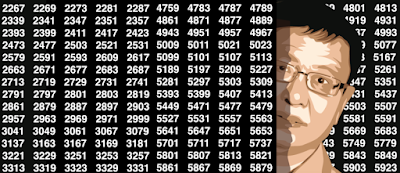## Thursday, January 11, 2018

### Beautiful Number Theory Problem and Sandbox for Data Scientists

The Waring conjecture - actually a problem associated with a number of conjectures, many now being solved - is one of the most fascinating mathematical problems. This article covers new aspects of this problem, with a generalization and new conjectures, some with a tentative solution, and a new framework to tackle the problem. Yet it is written in simple English and accessible to the layman.
I also review a number of famous related mathematical conjectures, including one with a \$1 million award still waiting for a solution, as well as Goldbach's conjecture, yet unproved as of today.  Many curious properties of the Floor function are also listed, and the emphasis is on machine learning and efficient computer-intensive algorithms to try to find surprising results, which then need to be formally proved or disproved.
1. General Framework
• Spectacular Result
• New Generalization of Golbach's Conjecture
• New Generalization of Fermat's Conjecture
2. Generalized Waring Problem
• Definitions
• Main Results
• Open Problems
• Fun Facts (Actually, Conjectures!)
3. Algorithms and Source Code
• Case n = 2: Sums of Two Terms
• Case n = 4: Sums of Four Terms
4. Related Conjectures and Solved Problems
• The One Million Dollar Conjecture